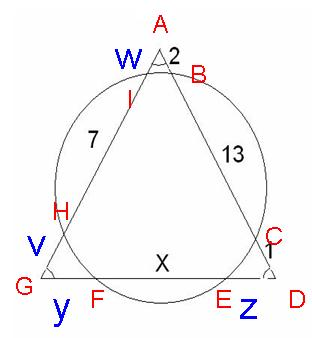# High School Problem?

## Recommended Posts

Where I work I have a patron who come in and picks my brain. Usually just politics, riddles or I.Q. Test type questions. Most I chew up and spit out rather quickly and haven't had a problem until now.

I admitted to him I was no mathematician but he gave me this problem stating it was only a high school problem, but it took him 2 months to figure out. I took a look at it and it seemed fairly easy but I can't seem to find the right equation or series of to solve for the unknowns. It's been a month and I've been scanning over all my old texts for similiar questions but to no avail.

Any help would be greatly appreciated, even just a general point in the right direction. I'd put some of my work up but quite frankly I'm embarrassed as it goes NOWHERE at all.EDIT: Sorry I forgot to add in case you can't tell from my diagram... that's a circle and it's overlapping an equilateral triangle. And you're trying to solve for X. Sorry again about the diagram.

##### Share on other sites

It's an equilateral triangle but the sides are different lengths...?

##### Share on other sites

It is an equilateral triangle but the numbers givin aren't the length of the whole side but rather the length of the part of that side which falls within the circle.

##### Share on other sites

Each side is 16 units.

You know that since the sum of the 3 values on the one side equal 16.

1 + 2 + 13 = 16

That's the easy part so the other sides are equal to:

16 - x

and

16 - 7

You can tell from the numbers the circle isn't centered which causes the problems.

##### Share on other sites

i think I can see how to do it, let me work through it

EDIT:

bleh I cba, and don't have time atm, will do it after my exams

##### Share on other sites

u can join the points where the circle cuts the triangle to the centre of the circle. Then u'll get 3 issoceles triangles in side the original triangle, n x would be the base of one of these issoceles triangles. And then....(i donnu the rest, still thinking)

##### Share on other sites

maybe there is some way to set up a porportion...

##### Share on other sitesi'll go ask my algeo techer##### Share on other sitesThe red letters are the intersection points of the triangle and the circle and the blue letters are variable for the unknown segments.

The length of each of the sides are the same since the triangle is an equilateral.

$AD = DG = GA = 16$

We will need that later.

We will start by solving for W:

We can use the theorem that says for two secant lines that intersect outside of a circle the product of the secant line and the exterior segment of one line are equal to the product of the secant line and exterior segment of the other line.

$(AH)(AI) = (AC)(AB)$

$(7+w)(w) = (15)(2)$

This gives us:$w=3$

since

$v+7+w=16$

$v+7+3=16$

$v=6$

using the same principle as above for the secant lines from the other angles of the triangle we get:

$(GH)(GI)=(GF)(GE)$

$(6)(13)=(y)(y+x)$

$y^2 +xy=78$ eqtn 1

and

$(DC)(DB)=(DE)(DF)$

$(1)(14)=(z)(z+x)$

$z^2 + xz =14$ eqtn 2

Solving the length of the side $x+y+z=16$

for z

$z=16-x-y$ eqtn 3

plug into eqtn 2

$(16-x-y)^2 + x(16-x-y) =14$

gives us

$y=10-\frac{x}{2}$

plug this into eqtn 3

$z=16-x-(10-\frac{x}{2})$

$z=16-x-10+\frac{x}{2}$

$z=6-\frac{x}{2}$

plug these eqtns for y into eqtns 1

$y^2 +xy=78$

$(10-\frac{x}{2})^2 +x(10-\frac{x}{2})=78$

gives us

$x=2 \sqrt{22}$

##### Share on other sites

I knew it was some weird rule I couldn't remember. Thanks for all your help, pepperchin. I appreciate the fact you explained your steps so I can clearly see how it's all done.

I guess it really was a high school problem, just to prove one theorem. I should probably go through my textbooks again just to remember all that I've forgotten.

I'll get back soon with the answer but that seems right to me, if that theorem is true.

##### Share on other sites

It is very true. I tutor high school kids in math so I have copies of the textbooks and it is a true theorem.

##### Share on other sites

Sorry I didn't mean to sound like I doubted you.

I'm sure it's correct, I just want to work through it myself and find some proofs of that theorem. I'm just quirky like that. I have to see it for myself.

I'm still impressed you have knowledge like that at your disposal. Thanks again for your help.

##### Share on other sites

This isn't a very tough problem but then, it is not a simple problem.

I think someone mentioned this already but you need to use Power of a Point Theorem.

http://www.cut-the-knot.org/pythagoras/PPower.shtml

Hope it helps.

## Create an account

Register a new account